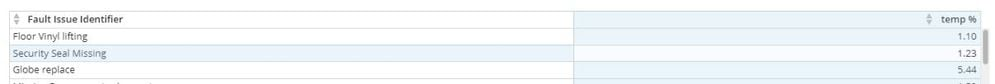# Pareto based on the higher percentage

Hi All,

i have data that is sorted by fault category (400+ categories) they are then sorted by the temperature (warm if greater than 24degC and cool if lower then 24degC) at the time of the fault.

What i would like to do is create a pareto based on the higher percentage of warm than cool. Any ideas?

• @Steve-DOMOnoob sorry for being dense, what is the math you're trying to do?

the pareto takes one axis and one measure...

so if you take

sum(CASE WHEN WARM ... then 1 else 0 END)

sum(CASE WHEN COLD ...  then 1 ELSE 0 END)

would that get the desired result?

• Thanks @jaeW_at_Onyx. As you have suggested i have used the below beast mode.

sum(CASE WHEN (CASE when`Temp`>=24 then 'warm' else 'cool' end)='WARM' then 1 else 0 END) / sum(CASE WHEN (CASE when`Temp`>=24 then 'warm' else 'cool' end)='COLD' then 1 ELSE 0 END)

this work perfectly to calculate the percentage. however when i look at the categories grouped it is adding the percentages. Any advice?• sum(CASE WHEN (CASE when`Temp`>=24 then 'warm' else 'cool' end)='WARM' then 1 else 0 END) / sum(CASE WHEN (CASE when`Temp`>=24 then 'warm' else 'cool' end)='COLD' then 1 ELSE 0 END)

... uh ... no ... it's not adding percentages.  it's just telling you that you have more Warm cases than cool cases.  i.e. you have 15 cool cases and 30 warm cases.

This discussion has been closed.